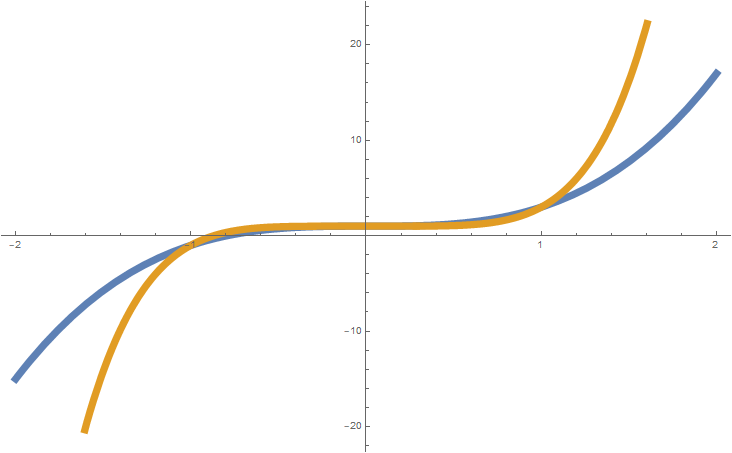Tuesday, October 20, 2020# PARCC algebra 2: write quotient as binomial

-

The following write-the-expression question, explained here in hopes of helping algebra 2 students in Maryland prepare for the PARCC test near the end of this school year, appears on the released version of the PARCC Algebra 2 sample items released following the 2016 test (#2).Plots of 2x3+1 (blue) and 2x5+1 (orange)

Given the expression$\frac{4n^{2t}-1}{2n^t-1}$

where t is an integer greater than or equal to 1,
write the expression as a binomial.

Solution strategy (there are others)$2n^t+1$

A different perspective:

When you have to rewrite the quotient of two binomials as a binomial, you can ask yourself: What binomial multiplied by the bottom binomial will give me the top binomial? In this problem, we have the question:$\textrm{?} \times (2n^t-1) = 4n^{2t}-1$

The multiplication of two binomials is just the FOIL rule, but how do we apply it in this case? The first term is easy:$\textrm{?} \times 2n^t = 4n^{2t}$

Since 2t = t + t and xn × xn = x2n, 2nt × 2nt = 4n2t. The first term in the binomial must then be 2nt.

Now, it seems the PARCC question writers have done you a favor with this and made the last term, which you can get from multiplying +1 with –1, very convenient for you. The resulting binomial is then$2n^t+1$

But that’s just because +1 times anything plus –1 times that same thing becomes zero. In this case, the “Outer” and “Inner” parts of our FOIL rule application just cancel each other out:$+2n^t-2n^t = 0$

If fully expanded, the multiplication would look like this:$(2n^t-1)(2n^t+1)$$= 4n^{2t}+2n^t-2n^t-1$$= 4n^{2t}-1$

## Analysis and resources for further study

Sal Khan, an engineer who launched the Khan Academy, discusses the FOIL rule as something mechanical you might just memorize. But, “when you’re 35, you’re not going to remember what FOIL means, and then you’re not going to be able to multiply this binomial,” he explains.

He then, more for commitment to long-term memory of how to multiply binomials, to explain the distributive property for multiplying binomials, which is also more logical, not a rote plug-and-play mnemonic.

The problem was perhaps a little easier than it needed to be to test this particular learning standard. As an additional challenge (if you’re game), write the binomial equivalent to the following expression:$\frac{16n^{2t}-64}{2n^t-4}$$8n^t+16$Paul Katulahttp://news.schoolsdo.org
Paul Katula is the executive editor of the Voxitatis Research Foundation, which publishes this blog. For more information, see the About page.

### Atlanta closes to in-person through year’s end

0
Public schools in Atlanta will continue e-learning through at least the end of December, due to a rise in Covid-19 cases in the community.